# fairnessMetrics

Bias and group metrics for a data set or classification model

Since R2022b

## Description

`fairnessMetrics` computes fairness metrics (bias and group metrics) for a data set or binary classification model with respect to sensitive attributes. The data-level evaluation examines binary, true labels of the data. The model-level evaluation examines the predicted labels returned by one or more binary classification models, using both true labels and predicted labels.

Bias metrics measure differences across groups, and group metrics contain information within the group. You can use the metrics to determine if your data or models contain bias toward a group within each sensitive attribute.

After creating a `fairnessMetrics` object, use the `report` function to generate a fairness metrics report or use the `plot` function to create a bar graph of the metrics.

## Creation

### Syntax

``evaluator = fairnessMetrics(SensitiveAttributes,Y)``
``evaluator = fairnessMetrics(Tbl,Y)``
``evaluator = fairnessMetrics(Tbl,ResponseName)``
``evaluator = fairnessMetrics(___,SensitiveAttributeNames=sensitiveAttributeNames)``
``evaluator = fairnessMetrics(___,Predictions=predictions)``
``evaluator = fairnessMetrics(___,Name=Value)``

### Description

````evaluator = fairnessMetrics(SensitiveAttributes,Y)` computes fairness metrics for the true, binary class labels in the vector `Y` with respect to the sensitive attributes in the `SensitiveAttributes` matrix. The `fairnessMetrics` function returns the `fairnessMetrics` object `evaluator`, which stores bias metrics and group metrics in the `BiasMetrics` and `GroupMetrics` properties, respectively.```
````evaluator = fairnessMetrics(Tbl,Y)` computes fairness metrics using the sensitive attributes in the table `Tbl` and the class labels in the vector `Y`.```
````evaluator = fairnessMetrics(Tbl,ResponseName)` computes fairness metrics using the sensitive attributes and response variable in the table `Tbl`. The input argument `ResponseName` specifies the name of the variable in `Tbl` that contains the class labels.```

example

````evaluator = fairnessMetrics(___,SensitiveAttributeNames=sensitiveAttributeNames)` specifies a subset of the variables in `Tbl` (whose names correspond to `sensitiveAttributeNames`) as sensitive attributes, or assigns names to the sensitive attributes in `sensitiveAttributeNames`. You can specify this argument in addition to any of the input argument combinations in the previous syntaxes.```

example

````evaluator = fairnessMetrics(___,Predictions=predictions)` computes fairness metrics for one or more binary classification models when you specify each model's predicted labels by using the `predictions` argument. `fairnessMetrics` uses both true labels and predicted labels for the model-level evaluation.```

example

````evaluator = fairnessMetrics(___,Name=Value)` specifies additional options using one or more name-value arguments. For example, specify `SensitiveAttributeNames="age",ReferenceGroup=30` to compute bias metrics for each group in the `age` variable with respect to the reference age group `30`.```

### Input Arguments

expand all

Sensitive attributes, specified as a vector or matrix. If you specify `SensitiveAttributes` as a matrix, each row of `SensitiveAttributes` corresponds to one observation, and each column corresponds to one sensitive attribute.

You can use the `sensitiveAttributeNames` argument to assign names to the variables in `SensitiveAttributes`.

Data Types: `single` | `double` | `logical` | `char` | `string` | `cell` | `categorical`

True, binary class labels, specified as a categorical, character, or string array; a logical or numeric vector; or a cell array of character vectors.

• `fairnessMetrics` supports only binary classification. `Y` must contain exactly two distinct classes.

• You can specify one of the two classes as a positive class by using the `PositiveClass` name-value argument.

• The length of `Y` must be equal to the number of observations in `SensitiveAttributes` or `Tbl`.

• If `Y` is a character array, then each label must correspond to one row of the array.

Data Types: `single` | `double` | `logical` | `char` | `string` | `cell` | `categorical`

Sample data, specified as a table. Each row of `Tbl` corresponds to one observation, and each column corresponds to one sensitive attribute. Multicolumn variables and cell arrays other than cell arrays of character vectors are not allowed.

Optionally, `Tbl` can contain columns for the true class labels, predicted class labels, and observation weights.

• You must specify the true class label variable using `ResponseName`, the predicted class label variables using `Predictions`, and the observation weight variable using `Weights`. `fairnessMetrics` uses the remaining variables as sensitive attributes. To use a subset of the remaining variables in `Tbl` as sensitive attributes, specify the variables by using `sensitiveAttributeNames`.

• The true class label variable must be a categorical, character, or string array, a logical or numeric vector, or a cell array of character vectors.

• `fairnessMetrics` supports only binary classification. The true class label variable must contain exactly two distinct classes.

• You can specify one of the two classes as a positive class by using the `PositiveClass` name-value argument.

• The column for the weights must be a numeric vector.

If `Tbl` does not contain the true class label variable, then specify the variable by using `Y`. The length of the response variable `Y` and the number of rows in `Tbl` must be equal. To use a subset of the variables in `Tbl` as sensitive attributes, specify the variables by using `sensitiveAttributeNames`.

Data Types: `table`

Name of the true class label variable, specified as a character vector or string scalar containing the name of the response variable in `Tbl`.

Example: `"trueLabel"` indicates that the `trueLabel` variable in `Tbl` (`Tbl.trueLabel`) is the true class label variable.

Data Types: `char` | `string`

Names of the sensitive attribute variables, specified as a character vector, string array of unique names, or cell array of unique character vectors. The functionality of `sensitiveAttributeNames` depends on the way you supply the sample data.

• If you supply `SensitiveAttributes` and `Y`, then you can use `sensitiveAttributeNames` to assign names to the variables in `SensitiveAttributes`.

• The order of the names in `sensitiveAttributeNames` must correspond to the column order of `SensitiveAttributes`. That is, `sensitiveAttributeNames{1}` is the name of `SensitiveAttributes(:,1)`, `sensitiveAttributeNames{2}` is the name of `SensitiveAttributes(:,2)`, and so on. Also, `size(SensitiveAttributes,2)` and `numel(sensitiveAttributeNames)` must be equal.

• By default, `sensitiveAttributeNames` is `{'x1','x2',...}`.

• If you supply `Tbl`, then you can use `sensitiveAttributeNames` to specify the variables to use as sensitive attributes. That is, `fairnessMetrics` uses only the variables in `sensitiveAttributeNames` to compute fairness metrics.

• `sensitiveAttributeNames` must be a subset of `Tbl.Properties.VariableNames` and cannot include the name of a class label variable or observation weight variable.

• By default, `sensitiveAttributeNames` is a set of all variable names in `Tbl`, except the variables specified by `ResponseName`, `Predictions`, and `Weights`.

Example: `SensitiveAttributeNames="Gender"`

Example: `SensitiveAttributeNames=["age","marital_status"]`

Data Types: `char` | `string` | `cell`

Predicted class labels (model predictions), specified as `[]`, the names of variables in `Tbl`, or a matrix.

Before R2023a: Specify predicted labels for at most one binary classifier by using the name of a variable in `Tbl` or a vector.

• `[]``fairnessMetrics` computes fairness metrics for the true class label variable (`Y` or the `ResponseName` variable in `Tbl`).

• Names of variables in `Tbl` — If you specify the input data as a table `Tbl`, then `predictions` can specify the name of one or more variables in `Tbl`, where each variable contains the predicted class labels for one model. In this case, you must specify `predictions` as a character vector, string array, or cell array of character vectors. For example, if the table contains one vector of class labels, stored as `Tbl.Pred`, then specify `predictions` as `"Pred"`.

• Matrix — Each row of the matrix corresponds to a sample, and each column corresponds to one model's predicted class labels. The number of rows in `predictions` must be equal to the number of samples in `Y` or `Tbl`. The predicted class labels in `predictions` must be elements of the true class label variable, and `predictions` must have the same data type as the true class label variable.

Note

If you specify predicted labels for one or more binary classification models, `fairnessMetrics` computes fairness metrics for each model that returned predicted labels.

Example: `Predictions="Pred"`

Example: `Predictions=["SVMPred","TreePred"]`

Data Types: `single` | `double` | `logical` | `char` | `string` | `cell` | `categorical`

Name-Value Arguments

Specify optional pairs of arguments as `Name1=Value1,...,NameN=ValueN`, where `Name` is the argument name and `Value` is the corresponding value. Name-value arguments must appear after other arguments, but the order of the pairs does not matter.

Example: `Predictions="P",Weights="W"` specifies the variables `P` and `W` in the table `Tbl` as the model predictions and observation weights, respectively.

Since R2023a

Names of the models with the predicted class labels `predictions`, specified as a character vector, string array of unique names, or cell array of unique character vectors.

• When `predictions` is an array of names in `Tbl`, the order of the names in `ModelNames` must correspond to the order of the names in `predictions`. That is, `ModelNames{1}` is the name of the model with predicted labels in the `predictions{1}` table variable, `ModelNames{2}` is the name of the model with predicted labels in the `predictions{2}` table variable, and so on. `numel(ModelNames)` and `numel(predictions)` must be equal. By default, the `ModelNames` value is equivalent to `predictions`.

• When `predictions` is a matrix, the order of the names in `ModelNames` must correspond to the column order of `predictions`. That is, `ModelNames{1}` is the name of the model with the predicted labels `predictions(:,1)`, `ModelNames{2}` is the name of the model with the predicted labels `predictions(:,2)`, and so on. `numel(ModelNames)` and `size(predictions,2)` must be equal. By default, the `ModelNames` value is `{'Model1','Model2',...}`.

• When `predictions` is `[]`, the `ModelNames` value is `'Model1'`.

Note

You cannot specify the `ModelNames` value when the `predictions` value is `[]`.

Example: `ModelNames="Ensemble"`

Example: `ModelNames=["SVM","Tree"]`

Data Types: `char` | `string` | `cell`

Label of the positive class, specified as a scalar. `PositiveClass` must have the same data type as the true class label variable.

The default `PositiveClass` value is the second class of the binary labels, according to the order returned by the `unique` function with the `"sorted"` option specified for the true class label variable.

Example: `PositiveClass=categorical(">50K")`

Data Types: `categorical` | `char` | `string` | `logical` | `single` | `double` | `cell`

Reference group for each sensitive attribute, specified as a numeric vector, string array, or cell array. Each element in the `ReferenceGroup` value must have the same data type as the corresponding sensitive attribute. If the sensitive attributes have mixed types, specify `ReferenceGroup` as a cell array. The number of elements in the `ReferenceGroup` value must match the number of sensitive attributes.

The default `ReferenceGroup` value is a vector containing the mode of each sensitive attribute. The mode is the most frequently occurring value without taking into account observation weights.

Example: `ReferenceGroup={30,categorical("Married-civ-spouse")}`

Data Types: `single` | `double` | `string` | `cell`

Observation weights, specified as a vector of scalar values or the name of a variable in `Tbl`. The software weights the observations in each row of `SensitiveAttributes` or `Tbl` with the corresponding value in `Weights`. The size of `Weights` must equal the number of rows in `SensitiveAttributes` or `Tbl`.

If you specify the input data as a table `Tbl`, then `Weights` can be the name of a variable in `Tbl` that contains a numeric vector. In this case, you must specify `Weights` as a character vector or string scalar. For example, if the weights vector `W` is stored in `Tbl.W`, then specify `Weights` as `"W"`.

Example: `Weights="W"`

Data Types: `single` | `double` | `char` | `string`

## Properties

expand all

Bias metrics, specified as a table.

`fairnessMetrics` computes the bias metrics for each group in each sensitive attribute, compared to the reference group of the attribute.

Each row of `BiasMetrics` contains the bias metrics for a group in a sensitive attribute.

• For data-level evaluation, the first and second variables in `BiasMetrics` correspond to the sensitive attribute name (`SensitiveAttributeNames` column) and the group name (`Groups` column), respectively.

• For model-level evaluation, the first variable corresponds to the model name (`ModelNames` column). The second and third variables correspond to the sensitive attribute name and the group name, respectively.

The rest of the variables correspond to the bias metrics in this table.

Metric NameDescriptionEvaluation Type
`StatisticalParityDifference`Statistical parity difference (SPD)Data-level or model-level evaluation
`DisparateImpact`Disparate impact (DI)Data-level or model-level evaluation
`EqualOpportunityDifference`Equal opportunity difference (EOD)Model-level evaluation
`AverageAbsoluteOddsDifference`Average absolute odds difference (AAOD)Model-level evaluation

The supported bias metrics depend on whether you specify predicted labels by using the `Predictions` argument when you create a `fairnessMetrics` object.

• Data-level evaluation — If you specify true labels and do not specify predicted labels, the `BiasMetrics` property contains only `StatisticalParityDifference` and `DisparateImpact`.

• Model-level evaluation — If you specify both true labels and predicted labels, the `BiasMetrics` property contains all metrics listed in the table.

For definitions of the bias metrics, see Bias Metrics.

Data Types: `table`

Group metrics, specified as a table.

The `fairnessMetrics` function computes the group metrics for each group in each sensitive attribute. Note that the function does not use the observation weights (specified by the `Weights` name-value argument) to count the number of samples in each group (`GroupCount` value). The function uses `Weights` to compute the other metrics.

Each row of `GroupMetrics` contains the group metrics for a group in a sensitive attribute.

• For data-level evaluation, the first and second variables in `GroupMetrics` correspond to the sensitive attribute name (`SensitiveAttributeNames` column) and the group name (`Groups` column), respectively.

• For model-level evaluation, the first variable corresponds to the model name (`ModelNames` column). The second and third variables correspond to the sensitive attribute name and the group name, respectively.

The rest of the variables correspond to the group metrics in this table.

Metric NameDescriptionEvaluation Type
`GroupCount`Group count, or number of samples in the groupData-level or model-level evaluation
`GroupSizeRatio`Group count divided by the total number of samplesData-level or model-level evaluation
`TruePositives`Number of true positives (TP)Model-level evaluation
`TrueNegatives`Number of true negatives (TN)Model-level evaluation
`FalsePositives`Number of false positives (FP)Model-level evaluation
`FalseNegatives`Number of false negatives (FN)Model-level evaluation
`TruePositiveRate`True positive rate (TPR), also known as recall or sensitivity, `TP/(TP+FN)`Model-level evaluation
`TrueNegativeRate`True negative rate (TNR), or specificity, `TN/(TN+FP)`Model-level evaluation
`FalsePositiveRate`False positive rate (FPR), also known as fallout or 1-specificity, `FP/(TN+FP)`Model-level evaluation
`FalseNegativeRate`False negative rate (FNR), or miss rate, `FN/(TP+FN)`Model-level evaluation
`FalseDiscoveryRate`False discovery rate (FDR), `FP/(TP+FP)`Model-level evaluation
`FalseOmissionRate`False omission rate (FOR), `FN/(TN+FN)`Model-level evaluation
`PositivePredictiveValue`Positive predictive value (PPV), or precision, `TP/(TP+FP)`Model-level evaluation
`NegativePredictiveValue`Negative predictive value (NPV), `TN/(TN+FN)`Model-level evaluation
`RateOfPositivePredictions`Rate of positive predictions (RPP), `(TP+FP)/(TP+FN+FP+TN)`Model-level evaluation
`RateOfNegativePredictions`Rate of negative predictions (RNP), `(TN+FN)/(TP+FN+FP+TN)`Model-level evaluation
`Accuracy`Accuracy, `(TP+TN)/(TP+FN+FP+TN)`Model-level evaluation

The supported group metrics depend on whether you specify predicted labels by using the `Predictions` argument when you create a `fairnessMetrics` object.

• Data-level evaluation — If you specify true labels and do not specify predicted labels, the `GroupMetrics` property contains only `GroupCount` and `GroupSizeRatio`.

• Model-level evaluation — If you specify both true labels and predicted labels, the `GroupMetrics` property contains all metrics listed in the table.

Data Types: `table`

Since R2023a

Names of the models with the predicted class labels `predictions`, specified as a character vector or cell array of unique character vectors. (The software treats string arrays as cell arrays of character vectors.)

The `ModelNames` name-value argument sets this property.

Data Types: `char` | `cell`

Label of the positive class, specified as a scalar. (The software treats a string scalar as a character vector.)

The `PositiveClass` name-value argument sets this property.

Data Types: `categorical` | `char` | `logical` | `single` | `double` | `cell`

Reference group, specified as a numeric vector or cell array. (The software treats string arrays as cell arrays of character vectors.)

The `ReferenceGroup` name-value argument sets this property.

Data Types: `single` | `double` | `cell`

Name of the true class label variable, specified as a character vector containing the name of the response variable. (The software treats a string scalar as a character vector.)

Data Types: `char`

Names of the sensitive attribute variables, specified as a character vector or cell array of unique character vectors. (The software treats string arrays as cell arrays of character vectors.)

The `sensitiveAttributeNames` argument sets this property.

Data Types: `char` | `cell`

## Object Functions

 `report` Generate fairness metrics report `plot` Plot bar graph of fairness metric

## Examples

collapse all

Compute fairness metrics for true labels with respect to sensitive attributes by creating a `fairnessMetrics` object. Then, create a table of fairness metrics by using the `report` function, and plot bar graphs of the metrics by using the `plot` function.

Load the sample data `census1994`, which contains the training data `adultdata` and the test data `adulttest`. The data sets consist of demographic information from the US Census Bureau that can be used to predict whether an individual makes over \$50,000 per year. Preview the first few rows of the training data set.

```load census1994 head(adultdata)```
``` age workClass fnlwgt education education_num marital_status occupation relationship race sex capital_gain capital_loss hours_per_week native_country salary ___ ________________ __________ _________ _____________ _____________________ _________________ _____________ _____ ______ ____________ ____________ ______________ ______________ ______ 39 State-gov 77516 Bachelors 13 Never-married Adm-clerical Not-in-family White Male 2174 0 40 United-States <=50K 50 Self-emp-not-inc 83311 Bachelors 13 Married-civ-spouse Exec-managerial Husband White Male 0 0 13 United-States <=50K 38 Private 2.1565e+05 HS-grad 9 Divorced Handlers-cleaners Not-in-family White Male 0 0 40 United-States <=50K 53 Private 2.3472e+05 11th 7 Married-civ-spouse Handlers-cleaners Husband Black Male 0 0 40 United-States <=50K 28 Private 3.3841e+05 Bachelors 13 Married-civ-spouse Prof-specialty Wife Black Female 0 0 40 Cuba <=50K 37 Private 2.8458e+05 Masters 14 Married-civ-spouse Exec-managerial Wife White Female 0 0 40 United-States <=50K 49 Private 1.6019e+05 9th 5 Married-spouse-absent Other-service Not-in-family Black Female 0 0 16 Jamaica <=50K 52 Self-emp-not-inc 2.0964e+05 HS-grad 9 Married-civ-spouse Exec-managerial Husband White Male 0 0 45 United-States >50K ```

Each row contains the demographic information for one adult. The information includes sensitive attributes, such as `age`, `marital_status`, `relationship`, `race`, and `sex`. The third column `flnwgt` contains observation weights, and the last column `salary` shows whether a person has a salary less than or equal to \$50,000 per year (`<=50K`) or greater than \$50,000 per year (`>50K`).

This example evaluates the fairness of the `salary` variable with respect to age. Group the `age` variable into four bins.

```ageGroups = ["Age<30","30<=Age<45","45<=Age<60","Age>=60"]; adultdata.age_group = discretize(adultdata.age, ... [min(adultdata.age) 30 45 60 max(adultdata.age)], ... categorical=ageGroups);```

Plot the counts of individuals in each class (`<=50K` and `>50K`) by age.

```figure gc = groupcounts(adultdata,["age_group","salary"]); bar([gc.GroupCount(1:2:end),gc.GroupCount(2:2:end)]) xticklabels(ageGroups) xlabel("Age Group") ylabel("Group Count") legend(["<=50K",">50K"]) grid on```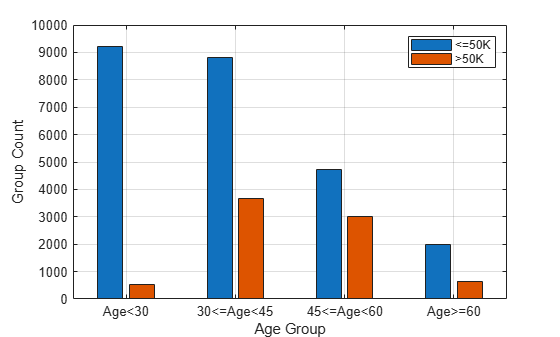Compute fairness metrics for the `salary` variable with respect to the `age_group` variable by using `fairnessMetrics`.

```evaluator = fairnessMetrics(adultdata,"salary", ... SensitiveAttributeNames="age_group",Weights="fnlwgt")```
```evaluator = fairnessMetrics with properties: SensitiveAttributeNames: 'age_group' ReferenceGroup: '30<=Age<45' ResponseName: 'salary' PositiveClass: >50K BiasMetrics: [4x4 table] GroupMetrics: [4x4 table] Properties, Methods ```

`evaluator` is a `fairnessMetrics` object. By default, the `fairnessMetrics` function selects the majority group of the sensitive attribute (group with the largest number of individuals) as the reference group for the attribute. Also, the `fairnessMetrics` function orders the labels by using the `unique` function with the `"sorted"` option, and specifies the second class of the labels as the positive class. In this data set, the reference group of `age_group` is the group `30<=Age<45`, and the positive class is `>50K`. `evaluator` stores bias metrics and group metrics in the `BiasMetrics` and `GroupMetrics` properties, respectively. Display the properties.

`evaluator.BiasMetrics`
```ans=4×4 table SensitiveAttributeNames Groups StatisticalParityDifference DisparateImpact _______________________ __________ ___________________________ _______________ age_group Age<30 -0.24365 0.17661 age_group 30<=Age<45 0 1 age_group 45<=Age<60 0.098497 1.3329 age_group Age>=60 -0.05041 0.82965 ```
`evaluator.GroupMetrics`
```ans=4×4 table SensitiveAttributeNames Groups GroupCount GroupSizeRatio _______________________ __________ __________ ______________ age_group Age<30 9711 0.29824 age_group 30<=Age<45 12489 0.38356 age_group 45<=Age<60 7717 0.237 age_group Age>=60 2644 0.081201 ```

According to the bias metrics, the `salary` variable is biased toward the age group 45 to 60 years and biased against the age group less than 30 years, compared to the reference group (`30<=Age<45`).

You can create a table that contains both bias metrics and group metrics by using the `report` function. Specify `GroupMetrics` as `"all"` to include all group metrics. You do not have to specify the `BiasMetrics` name-value argument because its default value is `"all"`.

`metricsTbl = report(evaluator,GroupMetrics="all")`
```metricsTbl=4×6 table SensitiveAttributeNames Groups StatisticalParityDifference DisparateImpact GroupCount GroupSizeRatio _______________________ __________ ___________________________ _______________ __________ ______________ age_group Age<30 -0.24365 0.17661 9711 0.29824 age_group 30<=Age<45 0 1 12489 0.38356 age_group 45<=Age<60 0.098497 1.3329 7717 0.237 age_group Age>=60 -0.05041 0.82965 2644 0.081201 ```

Visualize the bias metrics by using the `plot` function.

```figure t = tiledlayout(2,1); nexttile plot(evaluator,"spd") xlabel("") ylabel("") nexttile plot(evaluator,"di") xlabel("") ylabel("") xlabel(t,"Fairness Metric Value") ylabel(t,"Age Group")```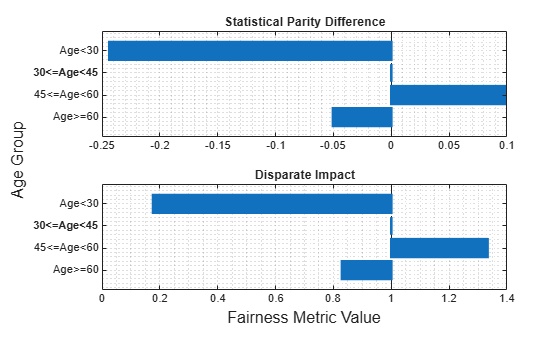The vertical line in each plot ($x=0$ for statistical parity difference and $x=1$ for disparate impact) indicates the metric value for the reference group. If the labels do not have a bias for a target group compared to the reference group, the metric value for the target group is the same as the metric value for the reference group.

Compute fairness metrics for predicted labels with respect to sensitive attributes by creating a `fairnessMetrics` object. Then, create a table of fairness metrics by using the `report` function, and plot bar graphs of the metrics by using the `plot` function.

Load the sample data `census1994`, which contains the training data `adultdata` and the test data `adulttest`. The data sets consist of demographic information from the US Census Bureau that can be used to predict whether an individual makes over \$50,000 per year. Preview the first few rows of the training data set.

```load census1994 head(adultdata)```
``` age workClass fnlwgt education education_num marital_status occupation relationship race sex capital_gain capital_loss hours_per_week native_country salary ___ ________________ __________ _________ _____________ _____________________ _________________ _____________ _____ ______ ____________ ____________ ______________ ______________ ______ 39 State-gov 77516 Bachelors 13 Never-married Adm-clerical Not-in-family White Male 2174 0 40 United-States <=50K 50 Self-emp-not-inc 83311 Bachelors 13 Married-civ-spouse Exec-managerial Husband White Male 0 0 13 United-States <=50K 38 Private 2.1565e+05 HS-grad 9 Divorced Handlers-cleaners Not-in-family White Male 0 0 40 United-States <=50K 53 Private 2.3472e+05 11th 7 Married-civ-spouse Handlers-cleaners Husband Black Male 0 0 40 United-States <=50K 28 Private 3.3841e+05 Bachelors 13 Married-civ-spouse Prof-specialty Wife Black Female 0 0 40 Cuba <=50K 37 Private 2.8458e+05 Masters 14 Married-civ-spouse Exec-managerial Wife White Female 0 0 40 United-States <=50K 49 Private 1.6019e+05 9th 5 Married-spouse-absent Other-service Not-in-family Black Female 0 0 16 Jamaica <=50K 52 Self-emp-not-inc 2.0964e+05 HS-grad 9 Married-civ-spouse Exec-managerial Husband White Male 0 0 45 United-States >50K ```

Each row contains the demographic information for one adult. The information includes sensitive attributes, such as `age`, `marital_status`, `relationship`, `race`, and `sex`. The third column `flnwgt` contains observation weights, and the last column `salary` shows whether a person has a salary less than or equal to \$50,000 per year (`<=50K`) or greater than \$50,000 per year (`>50K`).

Train a classification tree using the training data set `adultdata`. Specify the response variable, predictor variables, and observation weights by using the variable names in the `adultdata` table.

```predictorNames = ["capital_gain","capital_loss","education", ... "education_num","hours_per_week","occupation","workClass"]; Mdl = fitctree(adultdata,"salary", ... PredictorNames=predictorNames,Weights="fnlwgt");```

Predict the test sample labels by using the trained tree `Mdl`.

`labels = predict(Mdl,adulttest);`

This example evaluates the fairness of the predicted labels with respect to age and marital status. Group the `age` variable into four bins.

```ageGroups = ["Age<30","30<=Age<45","45<=Age<60","Age>=60"]; adulttest.age_group = discretize(adulttest.age, ... [min(adulttest.age) 30 45 60 max(adulttest.age)], ... categorical=ageGroups);```

Plot the counts of individuals in each predicted class (`<=50K` and `>50K`) by age.

```figure gs_age = groupcounts({adulttest.age_group,labels}); b_age = bar([gs_age(1:2:end),gs_age(2:2:end)]); xticklabels(ageGroups) xlabel("Age Group") ylabel("Group Count") legend(["<=50K",">50K"]) grid minor```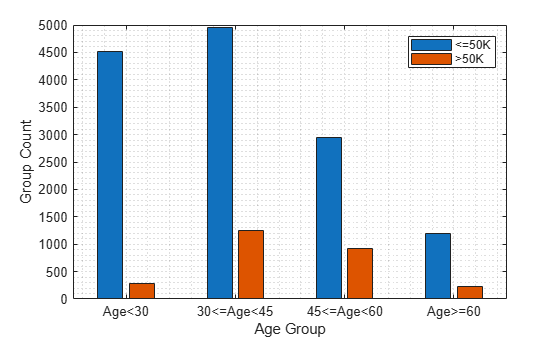Plot the counts of individuals by marital status. Display the count values near the tips of the bars if the values are smaller than 100.

```figure gs_status = groupcounts({adulttest.marital_status,labels}); b_status = bar([gs_status(1:2:end),gs_status(2:2:end)]); xticklabels(unique(adulttest.marital_status)) xlabel("Marital Status") ylabel("Group Count") legend(["<=50K",">50K"]) grid minor xtips1 = b_status(1).XEndPoints; ytips1 = b_status(1).YEndPoints; labels1 = string(b_status(1).YData); ind1 = ytips1 < 100; text(xtips1(ind1),ytips1(ind1),labels1(ind1), ... HorizontalAlignment="center",VerticalAlignment="bottom", ... Color=b_status(1).FaceColor) xtips2 = b_status(2).XEndPoints; ytips2 = b_status(2).YEndPoints; labels2 = string(b_status(2).YData); ind2 = ytips2 < 100; text(xtips2(ind2),ytips2(ind2),labels2(ind2), ... HorizontalAlignment="center",VerticalAlignment="bottom", ... Color=b_status(2).FaceColor)```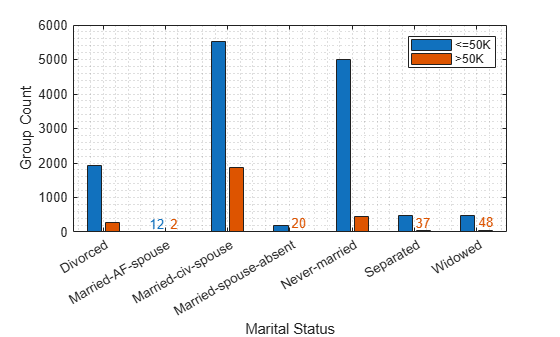Compute fairness metrics for the predictions (`labels`) with respect to the `age_group` and `marital_status` variables by using `fairnessMetrics`.

```MdlEvaluator = fairnessMetrics(adulttest,"salary", ... SensitiveAttributeNames=["age_group","marital_status"], ... Predictions=labels,Weights="fnlwgt")```
```MdlEvaluator = fairnessMetrics with properties: SensitiveAttributeNames: {'age_group' 'marital_status'} ReferenceGroup: {'30<=Age<45' 'Married-civ-spouse'} ResponseName: 'salary' PositiveClass: >50K BiasMetrics: [11x7 table] GroupMetrics: [11x20 table] ModelNames: 'Model1' Properties, Methods ```

`MdlEvaluator` is a `fairnessMetrics` object. By default, the `fairnessMetrics` function selects the majority group of each sensitive attribute (group with the largest number of individuals) as the reference group for the attribute. Also, the `fairnessMetrics` function orders the labels by using the `unique` function with the `"sorted"` option, and specifies the second class of the labels as the positive class. In this data set, the reference groups of `age_group` and `marital_status` are the groups `30<=Age<45` and `Married-civ-spouse`, respectively, and the positive class is `>50K`. `MdlEvaluator` stores bias metrics and group metrics in the `BiasMetrics` and `GroupMetrics` properties, respectively.

Create a table with fairness metrics by using the `report` function. Specify `BiasMetrics` as `["eod","aaod"]` to include the equal opportunity difference (EOD) and average absolute odds difference (AAOD) metrics in the report table. The `fairnessMetrics` function computes the two metrics by using the true positive rates (TPR) and false positive rates (FPR). Specify `GroupMetrics` as `["tpr","fpr"]` to include TPR and FPR values in the table.

```metricsTbl = report(MdlEvaluator, ... BiasMetrics=["eod","aaod"],GroupMetrics=["tpr","fpr"])```
```metricsTbl=11×7 table ModelNames SensitiveAttributeNames Groups EqualOpportunityDifference AverageAbsoluteOddsDifference TruePositiveRate FalsePositiveRate __________ _______________________ _____________________ __________________________ _____________________________ ________________ _________________ Model1 age_group Age<30 -0.041586 0.044576 0.41333 0.041053 Model1 age_group 30<=Age<45 0 0 0.45491 0.088618 Model1 age_group 45<=Age<60 0.061227 0.031446 0.51614 0.086954 Model1 age_group Age>=60 0.001949 0.0099106 0.45686 0.070746 Model1 marital_status Divorced 0.078378 0.043429 0.54262 0.075653 Model1 marital_status Married-AF-spouse 0.073013 0.078573 0.53726 0 Model1 marital_status Married-civ-spouse 0 0 0.46424 0.084133 Model1 marital_status Married-spouse-absent -0.06725 0.048036 0.39699 0.055311 Model1 marital_status Never-married 0.083467 0.054954 0.54771 0.057692 Model1 marital_status Separated 0.027103 0.026543 0.49135 0.058151 Model1 marital_status Widowed 0.12427 0.079864 0.58851 0.048675 ```

Plot the EOD and AAOD values for the sensitive attribute `age_group`. Because `age_group` is the first element in the `SensitiveAttributeNames` property of `MdlEvaluator`, it is the default value for the property. Therefore, you do not have to specify the `SensitiveAttributeName` argument of the `plot` function.

```figure t = tiledlayout(1,2); nexttile plot(MdlEvaluator,"eod") title("EOD") xlabel("") ylabel("") nexttile plot(MdlEvaluator,"aaod") title("AAOD") xlabel("") ylabel("") yticklabels("") xlabel(t,"Fairness Metric Value") ylabel(t,"Age Group")```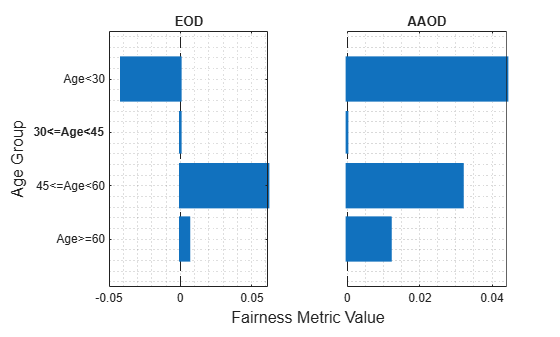The vertical line at $x=0$ indicates the metric value for the reference group (`30<=Age<45`). If the labels do not have a bias for a target group compared to the reference group, the metric value for the target group is the same as the metric value for the reference group. According to the EOD values (differences in TPR), the predictions for the `salary` variable are most biased toward the group `45<=Age<60` compared to the reference group. According to the AAOD values (averaged differences in TPR and FPR), the predictions are most biased toward the group `Age<30`.

Plot the EOD and AAOD values for the sensitive attribute `marital_status` by specifying the `SensitiveAttributeName` argument of the `plot` function as `marital_status`.

```figure t = tiledlayout(1,2); nexttile plot(MdlEvaluator,"eod",SensitiveAttributeName="marital_status") title("EOD") xlabel("") ylabel("") nexttile plot(MdlEvaluator,"aaod",SensitiveAttributeName="marital_status") title("AAOD") xlabel("") ylabel("") yticklabels("") xlabel(t,"Fairness Metric Value") ylabel(t,"Marital Status")```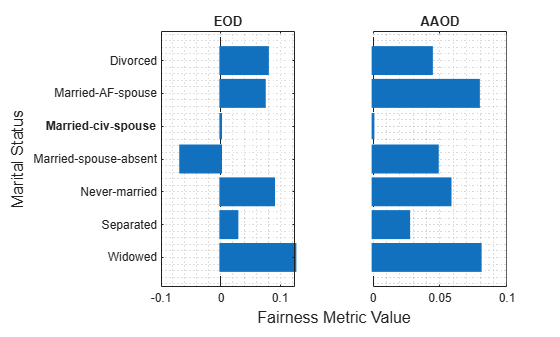The vertical line at $x=0$ indicates the metric value for the reference group (`Married-civ-spouse`). According to the EOD values, the predictions for the `salary` variable are most biased toward the group `Widowed` compared to the reference group. According to the AAOD values, the predictions are similarly biased toward the groups `Widowed` and `Married-AF-spouse`.

Train two classification models, and compare the model predictions by using fairness metrics.

Read the sample file `CreditRating_Historical.dat` into a table. The predictor data consists of financial ratios and industry sector information for a list of corporate customers. The response variable consists of credit ratings assigned by a rating agency.

`creditrating = readtable("CreditRating_Historical.dat");`

Because each value in the `ID` variable is a unique customer ID—that is, `length(unique(creditrating.ID))` is equal to the number of observations in `creditrating`—the `ID` variable is a poor predictor. Remove the `ID` variable from the table, and convert the `Industry` variable to a `categorical` variable.

```creditrating.ID = []; creditrating.Industry = categorical(creditrating.Industry);```

In the `Rating` response variable, combine the `AAA`, `AA`, `A`, and `BBB` ratings into a category of "good" ratings, and the `BB`, `B`, and `CCC` ratings into a category of "poor" ratings.

```Rating = categorical(creditrating.Rating); Rating = mergecats(Rating,["AAA","AA","A","BBB"],"good"); Rating = mergecats(Rating,["BB","B","CCC"],"poor"); creditrating.Rating = Rating;```

Train a support vector machine (SVM) model on the `creditrating` data. For better results, standardize the predictors before fitting the model. Use the trained model to predict labels and compute the misclassification rate for the training data set.

```predictorNames = ["WC_TA","RE_TA","EBIT_TA","MVE_BVTD","S_TA"]; SVMMdl = fitcsvm(creditrating,"Rating", ... PredictorNames=predictorNames,Standardize=true); SVMPredictions = resubPredict(SVMMdl); resubLoss(SVMMdl)```
```ans = 0.0872 ```

Train a generalized additive model (GAM).

```GAMMdl = fitcgam(creditrating,"Rating", ... PredictorNames=predictorNames); GAMPredictions = resubPredict(GAMMdl); resubLoss(GAMMdl)```
```ans = 0.0542 ```

`GAMMdl` achieves better accuracy on the training data set.

Compute fairness metrics with respect to the sensitive attribute `Industry` by using the model predictions for both models.

```predictions = [SVMPredictions,GAMPredictions]; evaluator = fairnessMetrics(creditrating,"Rating", ... SensitiveAttributeNames="Industry",Predictions=predictions, ... ModelNames=["SVM","GAM"]);```

Display the bias metrics by using the `report` function.

`report(evaluator)`
```ans=48×5 table Metrics SensitiveAttributeNames Groups SVM GAM ___________________________ _______________________ ______ _________ __________ StatisticalParityDifference Industry 1 -0.028441 0.0058208 StatisticalParityDifference Industry 2 -0.04014 0.0063339 StatisticalParityDifference Industry 3 0 0 StatisticalParityDifference Industry 4 -0.04905 -0.0043007 StatisticalParityDifference Industry 5 -0.015615 0.0041607 StatisticalParityDifference Industry 6 -0.03818 -0.024515 StatisticalParityDifference Industry 7 -0.01514 0.007326 StatisticalParityDifference Industry 8 0.0078632 0.036581 StatisticalParityDifference Industry 9 -0.013863 0.042266 StatisticalParityDifference Industry 10 0.0090218 0.050095 StatisticalParityDifference Industry 11 -0.004188 0.001453 StatisticalParityDifference Industry 12 -0.041572 -0.028589 DisparateImpact Industry 1 0.92261 1.017 DisparateImpact Industry 2 0.89078 1.0185 DisparateImpact Industry 3 1 1 DisparateImpact Industry 4 0.86654 0.98742 ⋮ ```

Among the bias metrics, compare the equal opportunity difference (EOD) values. Create a bar graph of the EOD values by using the `plot` function.

```b = plot(evaluator,"eod"); b(1).FaceAlpha = 0.2; b(2).FaceAlpha = 0.2; legend(Location="southwest")```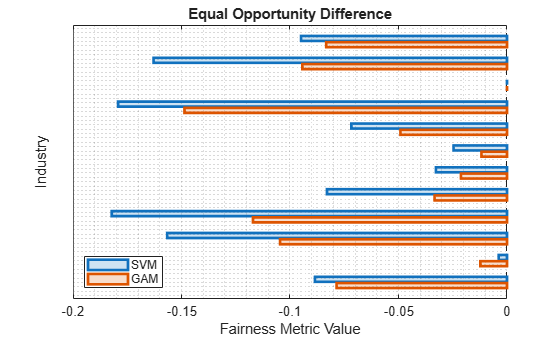To better understand the distributions of EOD values, plot the values using box plots.

```boxchart(evaluator.BiasMetrics.EqualOpportunityDifference, ... GroupByColor=evaluator.BiasMetrics.ModelNames) ax = gca; ax.XTick = []; ylabel("Equal Opportunity Difference") legend```The EOD values for GAM are closer to 0 compared to the values for SVM.

`fairnessMetrics` considers `NaN`, `''` (empty character vector), `""` (empty string), `<missing>`, and `<undefined>` values in `Tbl`, `Y`, and `SensitiveAttributes` to be missing values. `fairnessMetrics` does not use observations with missing values.